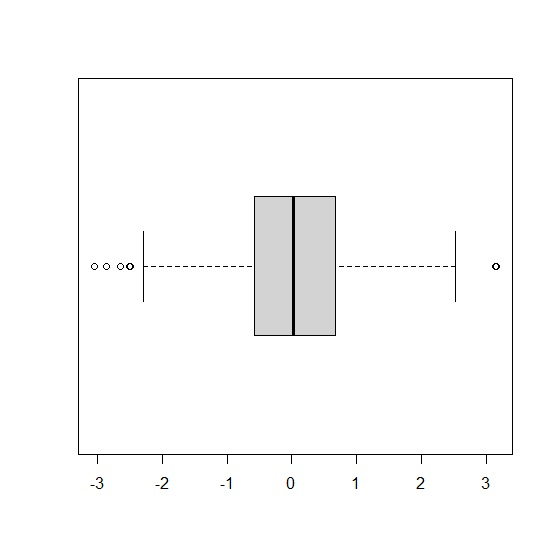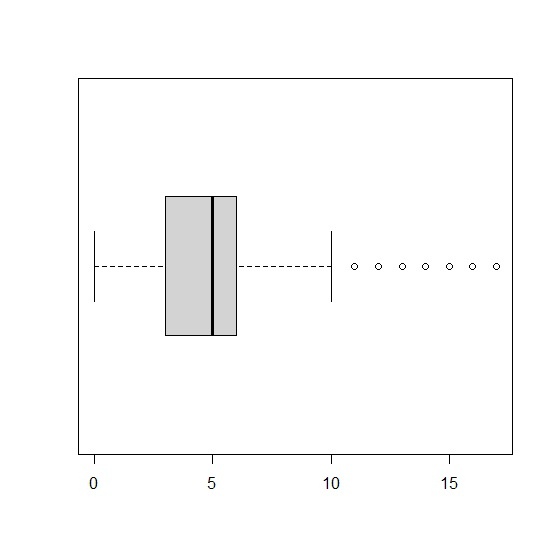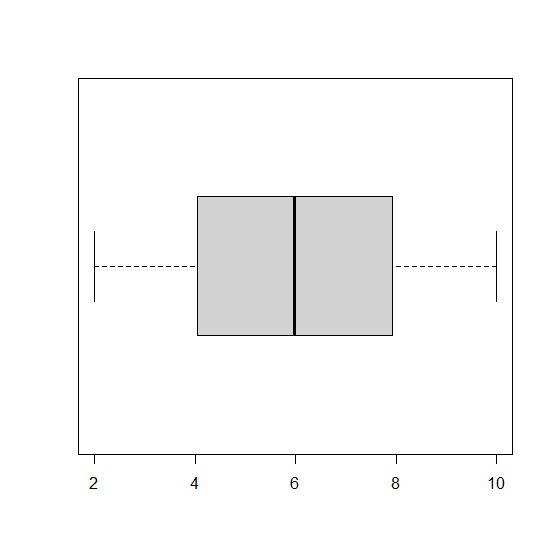# How to create a horizontal boxplot in base R?

To create a horizontal boxplot in base R, we can set the horizontal argument inside boxplot function to TRUE. For example, if we have a vector called x then the horizontal histogram of that vector can be created by using the command boxplot(x,horizontal=TRUE).

## Example1

x<-rnorm(500)
boxplot(x,horizontal=TRUE)

## Output## Example2

y<-rpois(10000,5)
boxplot(y,horizontal=TRUE)

## Output## Example3

z<-runif(1000,2,10)
boxplot(z,horizontal=TRUE)

## Output